Request a Free Counselling Session from our Expert Mentor# NCERT Solution for Class 10 Mathematics Chapter 2 Polynomials

In this chapter, you will learn about the fundamentals of polynomials and their applications in solving real-life problems. Polynomials are algebraic expressions consisting of variables and coefficients, which are used to represent complex mathematical relationships.
The chapter covers the definition and properties of polynomials, including degree, leading coefficient, and constant term. You will learn how to perform various operations on polynomials, such as addition, subtraction, multiplication, and division. The chapter also covers the factorization of polynomials using different methods such as factor theorem, remainder theorem, and synthetic division.
The NCERT Solutions for Class 10 Mathematics Chapter 2 – Experienced teachers with deep knowledge of the subject have prepared polynomials. The solutions are designed to help you understand the concepts and easily. The step-by-step explanations in the solutions will help you quickly solve the problems.
These NCERT solutions will be helpful for students preparing for their board exams and those who want to strengthen their understanding of the chapter. So, start exploring the chapter and improve your problem-solving

## Answers of Mathematics NCERT solutions for class 10 Chapter 2 Polynomials

Unit 2

Polynomials

Exercise 2.1

*Question 1:

The graphs of are given in following ﬁgure, for some polynomials p(x). Find the number of zeroes of p(x), in each case.

(i)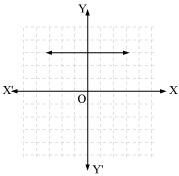(ii)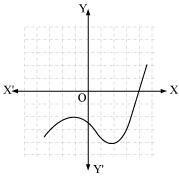(iii)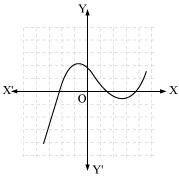(iv)

(v)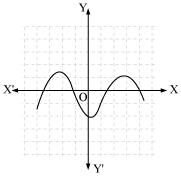(vi)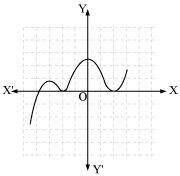1. The number of zeroes is 0 as the graph does not cut the x-axis at any point.
1. The number of zeroes is 1 as the graph intersects the x-axis at only 1 point.
1. The number of zeroes is 3 as the graph intersects the x-axis at 3 points.
1. The number of zeroes is 2 as the graph intersects the x-axis at 2 points.
1. The number of zeroes is 4 as the graph intersects the x-axis at 4 points.
1. The number of zeroes is 3 as the graph intersects the x-axis at 3 points.

Exercise 2.2

Question 1:

Find the zeroes of the following quadratic polynomials and verify the relationship between the zeroes and the coefficients.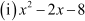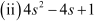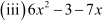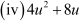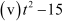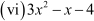Answer: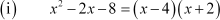The value ofis zero when i.e., when x = 4 or x = −2

Therefore, the zeroes ofare 4 and −2.

Sum of zeroes =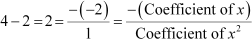Product of zeroes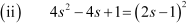The value of is zero when , i.e.,

Therefore, the zeroes of 4s2 − 4s + 1 are .

Sum of zeroes =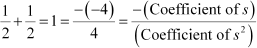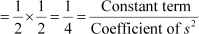Product of zeroes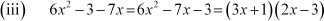The value of 6x2 − 3 − 7x is zero when

Therefore, the zeroes of

Sum of zeroes =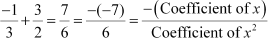Product of zeroes =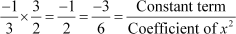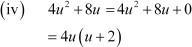The value of is zero when Therefore, the zeroes of are 0 and −2.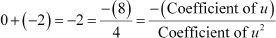Sum of zeroes =

Product of zeroes =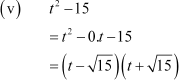The value of t2 − 15 is zero whenwhen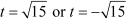Therefore, the zeroes of t2 − 15 are .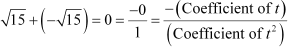Sum of zeroes =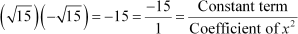Product of zeroes =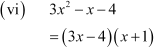The value of is zero when when orTherefore, the zeroes of

Sum of zeroes =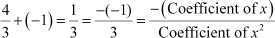Product of zeroes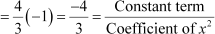*Question 2:

Find a quadratic polynomial each with the given numbers as the sum and product of its zeroes respectively.

Let the polynomial be and its zeroes be

(ii)

Let the polynomial be and its zeroes be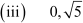Let the polynomial be and its zeroes be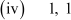Let the polynomial be and its zeroes be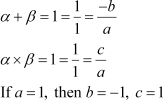Therefore, the quadratic polynomial is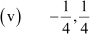Let the polynomial be and its zeroes be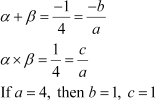Therefore, the quadratic polynomial isLet the polynomial be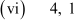Exercise 2.3

Question 1:

Divide the polynomial p(x) by the polynomial g(x) and ﬁnd the quotient and remainder in each of the following: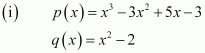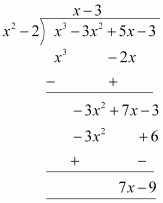Quotient = x − 3 Remainder = 7x − 9

Quotient = x2 + x − 3 Remainder = 8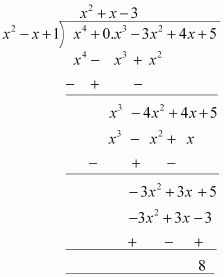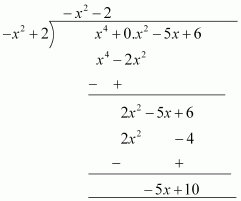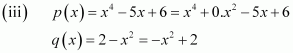Quotient = −x2 − 2

Remainder = −5x +10

Question 2:

Check whether the ﬁrst polynomial is a factor of the second polynomial by dividing the second polynomial by the ﬁrst polynomial: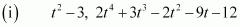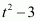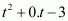=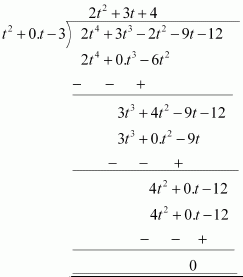Since the remainder is 0,

Hence,is a factor of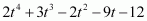.

Since the remainder is 0,

Hence, is a factor of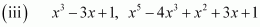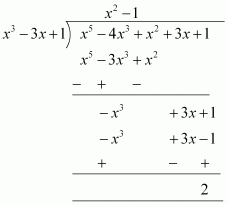Since the remainder

Hence,is not a factor of

*Question 3:

Obtain all other zeroes of if two of its zeroes are

Since the two zeroes are,

is a factor of

Therefore, we divide the given polynomial by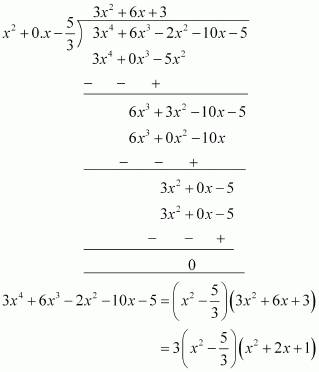We factorize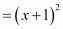Therefore, its zero is given by

As it has the term therefore, there will be 2 zeroes at x = −1.

Hence, the zeroes of the given polynomial areand −1.

*Question 4:

On dividingby a polynomial g(x), the quotient and remainder were x − 2 and − 2x + 4, respectively. Find g(x).

Answer: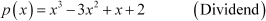g(x) = ? (Divisor)

Quotient

Remainder

Dividend = Divisor × Quotient + Remainder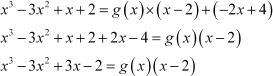g(x) is the quotient when we divideby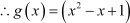Question 5:

Give examples of polynomial p(x), g(x), q(x) and r(x), which satisfy the division algorithm and

1. deg p(x) = deg q(x)
2. deg q(x) = deg r(x)
3. deg r(x) = 0

According to the division algorithm, if p(x) and g(x) are two polynomials with g(x) ≠ 0, then we can ﬁnd polynomials q(x) and r(x) such that

p(x) = g(x) × q(x) + r(x),

where r(x) = 0 or degree of r(x) < degree of g(x)

Degree of a polynomial is the highest power of the variable in the polynomial.

1. deg p(x) = deg q(x)

Degree of quotient will be equal to degree of dividend when divisor is constant ( i.e., when any polynomial is divided by a constant).

Let us assume the division of by 2.

Here,

g(x) = 2

Degree of p(x) and q(x) is the same i.e., 2. Checking for division algorithm,

p(x) = g(x) × q(x) + r(x)

Thus, the division algorithm is satisﬁed.

1. deg q(x) = deg r(x)

Let us assume the division of

Here,

Clearly, the degree of q(x) and r(x) is the same i.e., 1. Checking for division algorithm,

Thus, the division algorithm is satisﬁed. (iii)deg r(x) = 0

Degree of remainder will be 0 when remainder comes to a constant. Let us assume the division of x3 + 1by x2.

Here, p(x) = x3 + 1 g(x) = x2

q(x) = x and r(x) = 1

Clearly, the degree of r(x) is 0. Checking for division algorithm, p(x) = g(x) × q(x) + r(x)

x3 + 1 = (x2 ) × x + 1 x3 + 1 = x3 + 1

Thus, the division algorithm is satisﬁed.

Exercise 2.4

Question 1:

Verify that the numbers given alongside of the cubic polynomials below are their zeroes. Also verify the relationship between the zeroes and the coefficients in each case: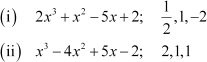(i)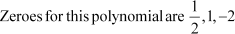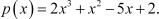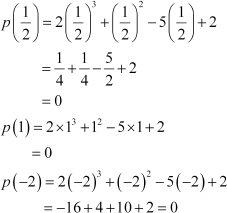Therefore,are the zeroes of the given polynomial.

Comparing the given polynomial withwe obtain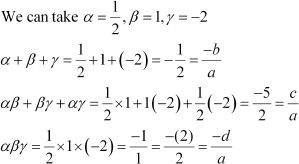Therefore, the relationship between the zeroes and the coefficients is veriﬁed.

(ii)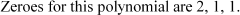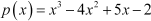Therefore, 2, 1, 1 are the zeroes of the given polynomial.

Comparing the given polynomial with , we obtain a = 1, b = −4, c = 5, d =−2.

Veriﬁcation of the relationship between zeroes and coefficient of the given polynomial

Multiplication of zeroes taking two at a time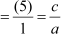Multiplication of zeroes

Hence, the relationship between the zeroes and the coefficients is veriﬁed.

Question 2:

Find a cubic polynomial with the sum of the product of its zeroes taken two at a time, and the product of its zeroes as 2, − 7, − 14 respectively.

Let the polynomial be and the zeroes bebe

It is given that

If a = 1, then b = −2, c = −7, d = 14

Hence, the polynomial is

Question 3:

If the zeroes of polynomialare ﬁnd a and b.

Answer: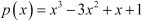Zeroes are a − b, a + a + b

Comparing the given polynomial with we obtain

p = 1, q = −3, r = 1, t = 1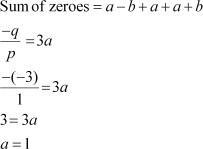The zeroes are

Hence, a = 1 and or

*Question 4:

If two zeroes of the polynomial are  ﬁnd other zeroes.

Given that andare zeroes of the given polynomial.

Therefore,

= x2 − 4x + 1 is a factor of the given polynomial

Clearly,

It can be observed that is also a factor of the given polynomial.

And

Therefore, the value of the polynomial is also zero when

Or x = 7 or −5

Hence, 7 and −5 are also zeroes of this polynomial.

*Question 5:

If the polynomialis divided by another polynomial         the remainder comes out to be x + a, ﬁnd k and a.

By division algorithm,

Dividend = Divisor × Quotient + Remainder

Dividend − Remainder = Divisor × Quotient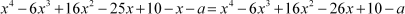will be perfectly divisible by

.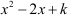Let us divideby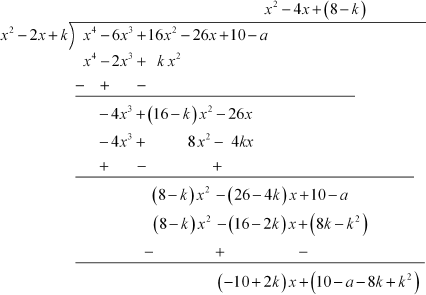It can be observed thatwill be 0.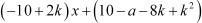Therefore,and

For

And thus, k = 5

For

Therefore, a = −5 Hence, k = 5 and a = −5

## NCERT Solutions for Class 10 Maths Chapter 2- Polynomials

As this is one of the important topics in maths, it comes under the unit – Algebra which has a weightage of 20 marks in the class 10 maths board exams. The average number of questions asked from this chapter is usually 1. This chapter talks about the following,

• Introduction to Polynomials
• Geometrical Meaning of the Zeros of Polynomial
• Relationship between Zeros and Coefficients of a Polynomial
• Division Algorithm for Polynomials

Polynomials are introduced in Class 9 where we discussed polynomials in one variable and their degrees in the previous class and this is discussed more in detail in Class 10. The NCERT solutions for class 10 mathematics for this chapter discusses the answers for various types of questions related to polynomials and their applications. We study the division algorithm for polynomials of integers and also whether the zeroes of quadratic polynomials are related to their coefficients.

The chapter starts with the introduction of polynomials in section 2.1 followed by two very important topics in section 2.2 and 2.3

• Geometrical Meaning of the zeroes of a Polynomial – It includes 1 question having 6 different cases.
• Relationship between Zeroes and Coefficients of a polynomial – Explore the relationship between zeroes and coefficients of a quadratic polynomial through solutions to 2 problems in Exercise 2.2 having 6 parts in each question.

Next, it discusses the following topics which were introduced in Class 9.

• Division Algorithm for Polynomials – In this, the solutions for 5 problems in Exercise 2.3 is given having three long questions.

### Key Features of NCERT Solutions for Class 10 Maths Chapter 2- Polynomials

• It covers the whole syllabus of Class 10 Maths.
• After studying through these NCERT solutions prepared by our subject experts, you will be confident to score well in exams.
• It follows NCERT guidelines which help in preparing the students accordingly.
• It contains all the important questions from the examination point of view.

Conclusion

The NCERT Solutions for Class 10 Mathematics Chapter 2 – Polynomials provides a comprehensive understanding of the fundamentals of polynomials and their applications in solving real-life problems. The chapter covers various topics, from the definition and properties of polynomials to factorization using different methods.
The solutions provided in this chapter have been designed to help students understand the concepts quickly and straightforwardly. The step-by-step approach in the solutions makes it easy for students to follow and understand each operation on polynomials.
These NCERT solutions will be helpful for students preparing for their board exams and those who want to strengthen their understanding of the chapter. By practicing the problems given in the NCERT solutions, students can improve their problem-solving skills and boost their confidence in the subject.
Overall, the NCERT Solutions for Class 10 Mathematics Chapter 2 – Polynomials are an excellent resource for students to improve their mathematical skills and gain a deeper understanding of polynomial expressions and their applications in real-life problems.

### Where can I get the accurate solution for NCERT Solution for Class 10 Maths Chapter 2?

At SWC’S you can get the accurate solution in PDF format for NCERT Solution for Class 10 Maths Chapter 2. The NCERT Textbook Solutions for the chapter Polynomials have been designed accurately by Mathematics experts at SWC’S. All these solutions are provided by considering the new pattern of CBSE, so that students can get thorough knowledge for their exams.

### Is it necessary to solve each problem provided in the NCERT Solution for Class 10 Maths Chapter 2?

Yes. Because these questions are important from an exam perspective. These questions are solved by experts to help the students to crack exercise very easily. These solutions help students to familiarize themselves with the polynomials. Solutions are available in PDF format on SWC’S website.

### List out the concepts covered in NCERT Solution for Class 10 Maths Polynomials?

The concepts are covered in NCERT Solution for Class 10 Mathematics Polynomials are introduction to polynomials, geometrical meaning of the zeros of polynomial, relationship between zeros and coefficients of a polynomial and division algorithm for polynomials.